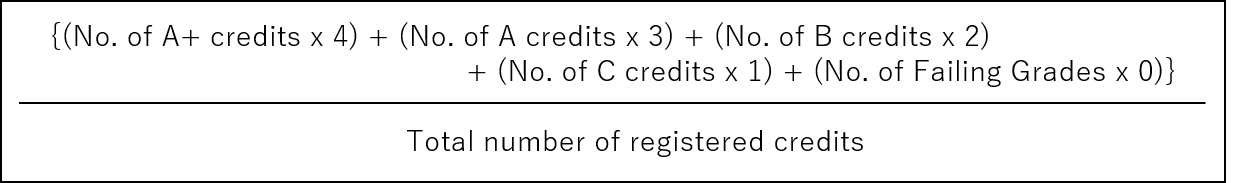# QI would like to know how to calculate my GPA.

A

Reference
GPA

## GPA Calculation Formula

A grade point average (GPA) is the sum of the product of the number of credits in a course and the grade point average (GPA). The GPA (Grade Point Average) is calculated by dividing the sum of the product of the number of credits in each course and the GradePoint by the total number of credits registered.*If you need an explanation of the GPA system at Waseda University, such as when entering a prospective employer or graduate school, please use the following explanatory documents as necessary.

Reference
Notification of the GPA System at Waseda University